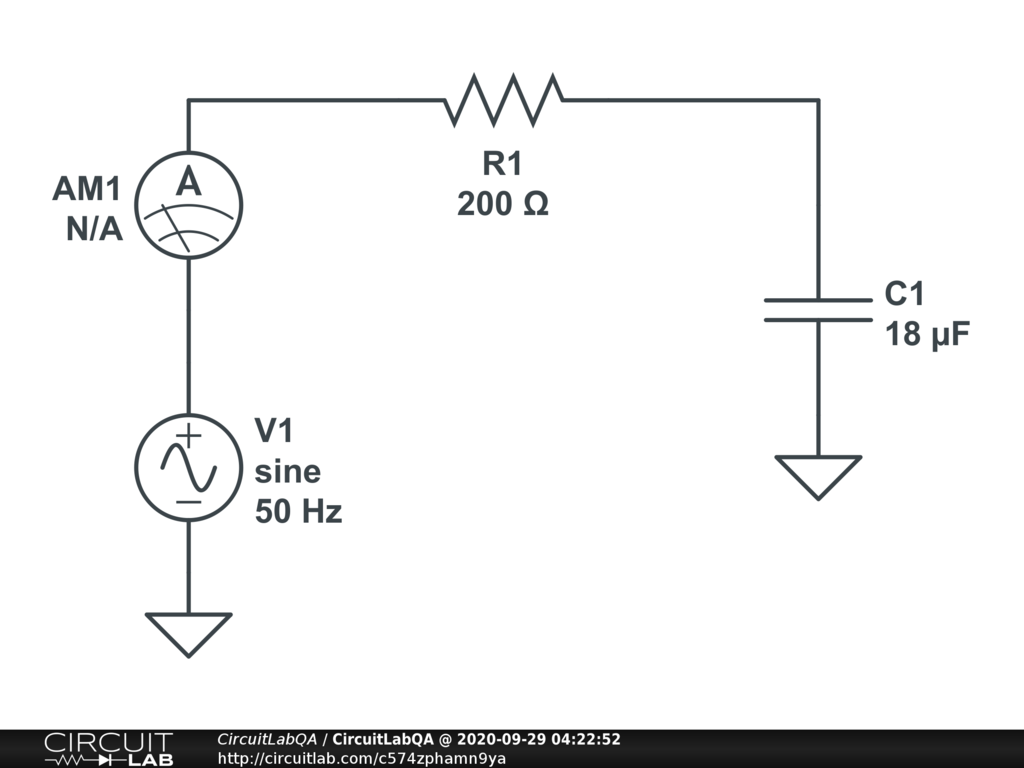## I'm having difficult calculating the capacitance when giving resistance, voltage, hertz, and output amps. Does anyone know how to solve this?

 The question reads: A resistor of 200 ohms and a capacitor of unknown value are connected to a 230 v, 50Hz supply resulting in a current of 0.85A flowing Calculate the value of the capacitor in micro Farads, and show all the working. Okay, so I came to the conclusion that the input current is 1.15 amps, but it says that the output current is 0.85A, how do I account for this with the equation? Thanks by Henrys September 28, 2020

## 1 Answer

### Answer by vanderghastWithout cap, you are right, it is 230 volt / 200 ohm = 1.15 amp. With a cap, the reactance is not just due to the resistor but also to the cap. The cap "resists" with 1 / (2 pi f C) and when combined with a resistor, both are added using Pythagoras' like theorem for a rigth triangle. So 0.85 amp = 230 volt / ( 200^2 + 1/( 6.28 * 50 hz * C)^2 )^0.5 solved for C gives C = 17. +/- microFarad. The closest standard cap value is 18 microFarad. (Given the usual cap. tolerance, that is the most likely "intended" value). +1 vote by vanderghast September 29, 2020 Technically, I should have use 325 volt p-p to get 230 volt rms but then, I would have not got 0.85 amp as easily spotted (or would have I ? ). Always embarrassing to get the most proper setting, with AC. by vanderghast September 29, 2020

## Your Answer

You must log in or create an account (free!) to answer a question.

### Ask a Question

Anyone can ask a question.

Did you already search (see above) to see if a similar question has already been answered? If you can't find the answer, you may ask a question.

### About This Site

CircuitLab's Q&A site is a FREE questions and answers forum for electronics and electrical engineering students, hobbyists, and professionals.

We encourage you to use our built-in schematic & simulation software to add more detail to your questions and answers.

Acceptable Questions:

• Concept or theory questions
• Practical engineering questions
• “Homework” questions
• Software/hardware intersection
• Best practices
• Design choices & component selection
• Troubleshooting

Unacceptable Questions:

• Non-English language content
• Non-question discussion
• Non-electronics questions
• Vendor-specific topics
• Pure software questions
• CircuitLab software support

Please respect that there are both seasoned experts and total newbies here: please be nice, be constructive, and be specific!

### About CircuitLab

CircuitLab is an in-browser schematic capture and circuit simulation software tool to help you rapidly design and analyze analog and digital electronics systems.